[ HOME ]  [ NEXT ]] [ BACK ]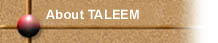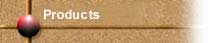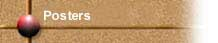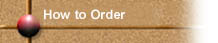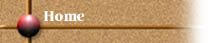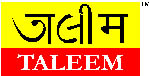A       D I V I S I O N      O F      E K L A V Y A      E D U C A T I O N      F O U N D A T I O N

 Code : TLM/M/017 Age : 9 - 11 yrs. Name: Binomial Cubes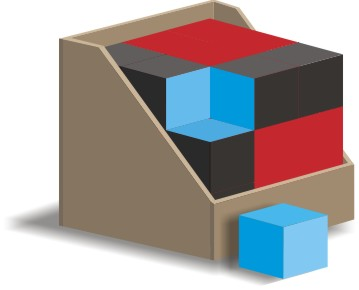The Binomial Cube is a concrete representation of the algebric formula (a+b)3. The Solution of the equation is a3 + 3ab2 + 3a1b + b2. The arrangement of various cubes and cuboids follow the pattern that explains the logic. Here, both the cubes have volume of a3 and b3 respectively. One set of three cuboids has volume of a2b and the other set has volume of a2b and the other set has volume of ab2. Together, they all represent the complete solution a3+3(ab2)+3(a2b)+b3. The Binomial Cube is contained in an eco-friendly corrugated box with a corrugated lid.

[ HOME ]  [ NEXT ]] [ BACK ]